NEET  >  Revision Notes: Laws of Motion & Friction

# Revision Notes: Laws of Motion & Friction - Notes | Study NCERT Exemplar & Revision Notes for NEET - NEET

 1 Crore+ students have signed up on EduRev. Have you?

MOMENTUM
Momentum of a particle (p) is equal to the mass of the particle (m) times velocity of the particle (v). So, p = mv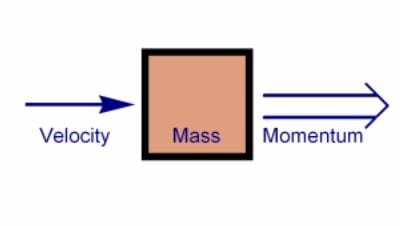Question for Revision Notes: Laws of Motion & Friction
Try yourself:Two trains A and B are running in the same direction on parallel tracks such that A is faster than B. If packets of equal weight are exchanged between the two, then

NEWTON LAWS OF MOTION
(i) First Law (Law of Inertia): Everybody continues in its state of rest or of uniform motion in a straight line unless it is compelled by some external force to change that state.
(ii) Second Law: The rate of change of momentum of a body is directly proportional to the impressed force and takes place in the direction of the force.
So, F = dp/dt
This results force acting a body F is equal to the mass of the body m times acceleration of the body a.
So, F = ma
(iii) Third Law: To Every action there is an equal and opposite reaction.
FAB = - FBA

• Impulse: Impulse of a force is defined as the change in momentum produced by the force and it is equal to the product of force and the time for which it acts.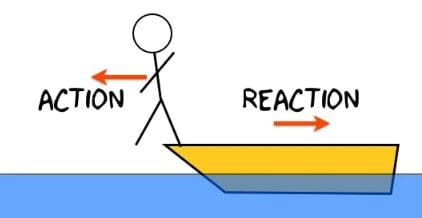So,  J = Ft
= mv – mu

• Apparent weight of a man inside a lift: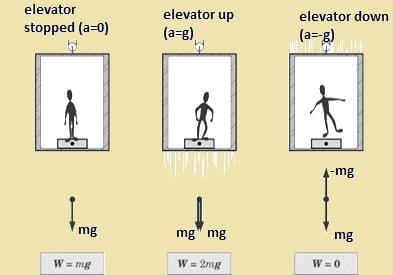(a) The lift possesses zero acceleration (fig-1): W = m
(b) The lift moving upward with an acceleration a (fig-2):
W = mg + ma
W= mg + mg
W= 2 mg
(c) The lift moving downward with an acceleration a (fig-3):
W = mg – ma
W = mg – mg
W = 0

• Inertial mass (mi)- It is defined as the ratio between force F to the acceleration a a produced in the body.
So, mi = F/a

NOTE
When the velocity of a body is comparable to the velocity of light, inertial mass changes with velocity in accordance with following formula,
mi = mi0/√(1-v2/c2)
Here mi0 is the rest mass of body, v is the velocity of body and c is the velocity of light.

• Conservation of linear momentum:
In an isolated system (no external force), the algebraic sum of the momentum of bodies, along any straight line, remains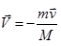constant and is not changed due to their mutual action and reaction on each other.
• Recoil of gun: If m is the mass of the bullet, M is the mass of gun and v is the mass of the bullet, then, velocity of the recoil of gun  V will be,
• Equilibrium of concurrent forces:
Forces are said to be concurrent if they meet at a common point. Below figure shows three concurrent forces ,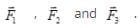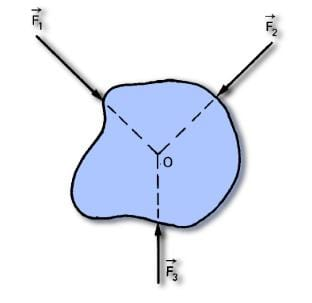• Moment of the force = F×d
Here F is the force and d is the distance of line of action.
• Momentum of a couple (Torque (τ)): Momentum of a couple is equal to the product of force F and the perpendicular distance r between them.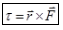• Work done by a couple: (W)
W = τ × θ
Here θ is the angular displacement.

• Connected Motion (Pulley Problem)
(a) Driven body moving vertically:
Acceleration of the system, a = (M-m/M+m) g
Tension in the string, T = (2Mm/M+m) g
The force on the pulley, F = (4Mm/M+m) g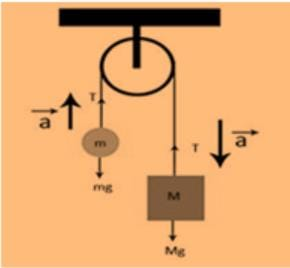(b) Driven body moving horizontally:
Acceleration of the system, a = (M /M+m) g
Tension in the string, T= (Mm/M+m) g
The force on the pulley, F= [√2 (Mm) /M+m] g
Here g is the free fall acceleration.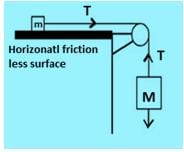• Application of Newton’s third law on contact of two block:
(a) Acceleration, a =F/(m + M)
(b)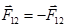(c) Contact force, F12 = (m/m+M) F = F21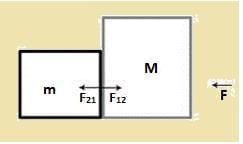• Friction: Whenever a body tends to slide over another’s surface, an opposing force, called force of friction comes into play. This force acts tangentially to the interface of two bodies.
• Static friction: Static friction is the force of friction between two surfaces so long as there is no relative motion between them. It is always equal to the applied force. The static frictional forces are incorporated in the following inequality.
fs  ≤ µsN
• The magnitude of static friction fs (static frictional force) has a maximum value fs,max that is given by,
fs,max  = µsN
Here µs is the coefficient of kinetic friction and N is the normal force.
So, coefficient of static friction, µs = fs,max /N
• Kinetic friction: Kinetic friction is the force of friction which comes into play between two surfaces when there is some relative motion between them. The magnitude of force of kinetic friction f(kinetic frictional force) is proportional to the normal force N.
So, fk = µk N
Here µk is the coefficient of kinetic friction.
Thus, coefficient of kinetic friction, µk = fk/N
• µk < µs
• Rolling friction: Force of friction which comes into play, between two surfaces, while one is rolling over the other is called rolling friction.  Rolling friction is similar to kinetic friction.
So, f= µN
Here µr is the coefficient of rolling friction and N is the normal force.
Question for Revision Notes: Laws of Motion & Friction
Try yourself:Maximum value of frictional force is called
• Angle of Friction:
The angle made by the resultant reaction force with the vertical (normal reaction) is known as the angle of the friction.
Now,  in the triangle OAB
AB/OB = cotθ
So, OB = AB/cotθ
= AB tanθ
Or, tanθ = OB/AB
= f/N
So, tanθ = f/N  = µs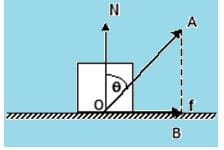• Angle of Repose: It is the angle which an inclined plane makes with the horizontal so that a body placed over it just begins to slide of its own accord.
Consider a body of mass m resting on an inclined plane of inclinationq. The forces acting on the body are shown – Ff being the force of friction. If friction is large enough, the body will not slide down.
along x: mg sin θ – f = 0             …(1)
Along y: N –mg cosθ = 0                …(2)
i.e. N = mg cos θ and f = mg sin θ
Thus,  gives,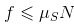mg sin θ ≤  μS mg cos θ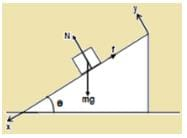So,  tan θ ≤ μS. This signifies, the coefficient of static friction between the two surfaces, in order that the body doesn’t slide down.
When q is increased, then tan θ > μ. Thus sliding begins, and the angle θr  = tan-1μ. This angle is known as the angle of repose.
• Acceleration on a horizontal plane: a = (F - µkN)/m
• If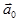be the acceleration of frame, the pseudo force will be,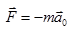(Example: centrifugal force = mv2/r = mrω2)
• Gravitational mass (mg):
mg = F /g
= FG r2/GM
Here, g is the free fall acceleration, FG is the gravitational force and G is the gravitational constant.
The document Revision Notes: Laws of Motion & Friction - Notes | Study NCERT Exemplar & Revision Notes for NEET - NEET is a part of the NEET Course NCERT Exemplar & Revision Notes for NEET.
All you need of NEET at this link: NEET

## NCERT Exemplar & Revision Notes for NEET

291 docs
 Use Code STAYHOME200 and get INR 200 additional OFF

## NCERT Exemplar & Revision Notes for NEET

291 docs

### How to Prepare for NEET

Read our guide to prepare for NEET which is created by Toppers & the best Teachers

Track your progress, build streaks, highlight & save important lessons and more!

,

,

,

,

,

,

,

,

,

,

,

,

,

,

,

,

,

,

,

,

,

;